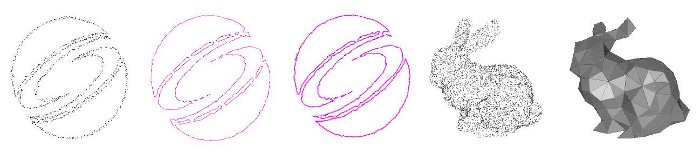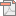english >> deutsch Home | UdS | MPI | SB

# selected publications_

### A Bayesian Approach to Manifold Topology Reconstruction#### Abstract

In this paper, we investigate the problem of statistical reconstruction of piecewise linear manifold topology. Given a noisy, probably undersampled point cloud from a one- or two-manifold, the algorithm reconstructs an approximated most likely mesh in a Bayesian sense from which the sample might have been taken. We incorporate statistical priors on the object geometry to improve the reconstruction quality if additional knowledge about the class of original shapes is available. The priors can be formulated analytically or learned from example geometry with known manifold tessellation. The statistical objective function is approximated by a linear programming / integer programming problem, for which a globally optimal solution is found. We apply the algorithm to a set of 2D and 3D reconstruction examples, demon-strating that a statistics-based manifold reconstruction is feasible, and still yields plausible results in situations where sampling conditions are violated.

Keywords: Surface reconstruction, topology reconstruction, machine learning and graphics, linear programmingRead Paper (~0.7MB) - 3160 times clicked

#### Bibliography

A. Tevs, M. Wand, I. Ihrke, H.-P. Seidel "A Bayesian Approach to Manifold Topology Reconstruction" , Research Report, Max Planck Institue Informatik, 2010, pages 1-23 [bibtex]